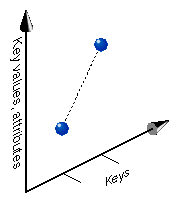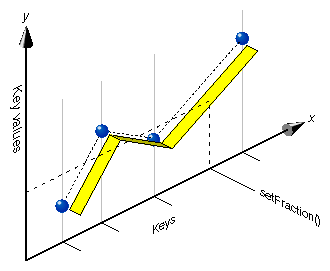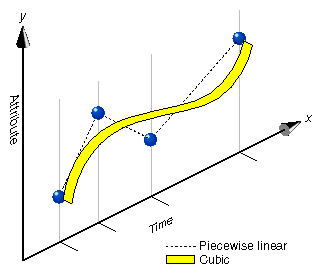## Chapter 10. Scene Graph Engines

There are classes that work with scene graphs, but are not nodes; they cannot be part of the scene graph, but they serve the vital function of enabling animation. csEngine is such a class.

This chapter describes csEngine and the multiple subclasses derived from it.

These are the sections in this chapter:

## Engines

A csEngine performs a specified function on input data and outputs a result. These results can be used, for example, to encapsulate scene graph behavior. If part of a scene graph renders a car, you might, attach an engine to the transform node of each wheel to animate its rotation. You might also tie the motion of the wheels to the motion of other shapes in the scene so they appear to pass by when the car moves, or you might cycle the car through a set of colors repeatedly.

csEngine is a derivative of csNode, but usually csEngines are not included as nodes in a scene graph.

### Input and Output Fields

csEngine has input and output fields. csEngine updates its output fields according to the function carried out by the csEngine. For example, a simple engine might take two inputs and output the average of the two.

csEngine updates its output fields only under the following conditions:

• Input fields have changed since the output fields were last read.

For example, the input fields might change twice, but if the output fields are read only after the second change in the input fields, the output fields are updated only once.

### Connecting Engines to Other Nodes

You connect an engine to other nodes or engines to create an animation, to cause a chain reaction of motions, or to cycle through a set of attributes, such as color cycling. You use csContainer::connect() to make the connections. For example, to make a color interpolator engine change a specific field in a material, connect the engine's output field value to the specific field, as follows:

 ```csColorInterpolator* myEngine = new csColorInterpolator; csMaterial* rose = new csMaterial; myEngine->connect(csColorInterpolator::VALUE, rose, csMaterialDIFFUSE_COLOR); ```

In this example, myEngine is connected to the color field of the rose object.

#### Connecting Engines to Other Engines

The output of one engine may also be connected to the input of another engine. For example, the output value of a csSpline might be connected to the input field of a csMorphEng, as follows:

 ```csMorphEng3f *morphEngine = new csMorphEng3f; csSpline *splineEngine = new csSpline; splineEngine->connect(csSpline::WEIGHT, morphEngine, csMorphEng3f::WEIGHT); ```

### Engine Types

Many engines interpolate between two values at specified increments. For example, a rotation interpolator might take the beginning and ending rotation coordinates and the incremental changes between the two. Its output might be a series of transformations that move an object through a series of coordinates that rotate it from the beginning to the ending coordinates.

Another example for using an engine is color cycling. You might take an interpolator engine that takes a series of colors as inputs and outputs a color that gradually changes to from one input color to the next.

The following csEngines interpolate data:

• csSpline—interpolates an arbitrary, non-uniform spline and outputs a weighted array that defines a weight for each key in the spline.

• csSelectorEng—selects one coordinate from an array input as its single output. Derived classes include csSelectorEng3f and csSelectorEng4f.

• csInterpolator—interpolates between keyframe values selected from the key array by a floating point fraction, ranging from 0 to 1.

• csColorInterpolator—linearly interpolates among a set of colors.

• csCoordinateInterpolator—linearly interpolates among sets of coordinates, possibly generating more than one output to help produce, for example, a geometric morph.

• csNormalInterpolator—linearly interpolates among a set of normal vectors, possibly generating more than one output to help produce, for example, a geometric morph.

• csOrientationInterpolator—linearly interpolates among a set of rotations.

• csPositionInterpolator—linearly interpolates among a set of positions in space.

• csScalarInterpolator—linearly interpolates between a set of floats.

csInterpolator is the base class for the following engines:

• csColorInterpolator

• csCoordinateInterpolator

• csNormalInterpolator

• csOrientationInterpolator

• csPositionInterpolator

• csScalarInterpolator

The following csEngines can be used to change the features of a shape:

• csMorphVec—produces a weighted sum of attribute sets. Derived classes include csMorphVec3f and csMorphVec4f.

• csTransformEng—transforms attribute sets consisting of points or vectors (homogeneous coordinate implicitly 1 or 0 respectively). Derived classes include csTransformEng3f is derived from csTransformEng.

The following sections describe each of these nodes.

## Engines that Interpolate Values

csInterpolator is an abstract base class that interpolates between keys, as shown in Figure 10-1. The keys might be location, normal, or rotation values.

Figure 10-1. Keys and Key ValuesInterpolator nodes are designed for linear, keyframed animation, that is, an interpolator node defines a piecewise linear function, f(k), on the interval [-∞, ∞]. The piecewise linear function is defined by n keys and n corresponding key values, f(k). The keys must be monotonic and non-decreasing. An interpolator node evaluates f(k) given any value of k.

### Interpolator Engine Terminology

Interpolator engines take one or more input values, perform a function on them, and output a result. The relationship between the input and output values can be represented by a graph where the x axis represents the input values and the y axis represents the output values. Graphing the points (xn, yn) shows the shape of the engine's function.

Interpolator engine methods use the following terminology:

• keys—input values represented on the x axis.

• key values—output values represented on the y axis.

• setFraction()—a method in interpolator engines that specifies a point on the y axis.

Figure 10-2 illustrates these terms.

Figure 10-2. Engine TerminologycsInterpolator Fields

The fields in csInterpolator include:

 ```void setFraction(float fraction); float getFraction(); csMFFloat* key(); ```

For an explanation of key() and setFraction(), see, “Interpolator Engine Terminology”.

### csSpline

A csSpline takes an array of keys as its input and outputs a set of weights. A key is one or more pieces of data; a weight is a fractional scaling factor. How the output is weighted depends on the order of the spline. Figure 10-3 shows two different splines that use the same key values, but are of different orders: piecewise linear and cubic.

Figure 10-3. SplineNote: Release 1.0 and 1.1 of Cosmo 3D only support the piecewise linear order.

Typically, the output of a csSpline is used as the input of a csMorphEng.

#### Keys and Key Values

In Figure 10-3, the X axis represents keys, such as time, and the Y axis represents any attribute or set of attributes, such as location, color, or transparency. Y axis values are called key values. Notice that the interval between the key values is nonuniform. After setting the key values, csSpline fills in the values between the key values.

The difference between the piecewise linear and cubic splines is created by the different weight factors.

Values outside of the maximum and minimum keys are clamped to the maximum and minimum keys and key values.

Key values, such as colors or coordinates, are not kept in a csSpline object. Consequently, a single csSpline object can define the animation spline for many keys. Key values are typically kept in a csMorphEng engine, which calculates the weighted sum of key values to produce the final result.

#### csSpline Fields

The following fields set the spline values:

 ```csMFFloat* key() const; csMFFloat*  weight() const; void setFraction(csFloat fraction); csFloat getFraction(); void setBasis(const csMatrix4f& basis); void getBasis(csMatrix4f& basis); ```

For an explanation of key() and setFraction(), see, “Interpolator Engine Terminology”.

weight() specifies the multiplication factor that changes the linear graph into a spline curve.

setBasis() specifies the matrix by which you multiply the four key values (closest to the fraction) to get the weight values.Note: setBasis() is ignored in Cosmo 3D release 1.0 and 1.1. All splines are piecewise linear.

#### csColorInterpolator

This node interpolates among a set of key values in a csMFColor to produce a csSFColor (RGB) color.

csColorInterpolator contains the following fields:

 ```csMFVec3f* keyValue() const; csSFVec3f value ```

keyValue() is the set of RGB color values over which you want to interpolate. The number of colors in the keyValue() field must be equal to the number of keyframes in the key field.

For a further explanation of keyValue(), see “Interpolator Engine Terminology”.

#### csCoordinateInterpolator

This node linearly interpolates among a set of MFVec3f values. This would be appropriate for interpolating coordinate positions for a geometric morph.

csCoordinateInterpolator contains the following fields:

 ```csMFVec3f* keyValue() const; csMFVec3f value ```

keyValue() contains sets of positions used for the coordinate interpolation. The number of elements stored in the value field is equal to the number of elements in the keyValue() field divided by the number of elements in the key field.

For a further explanation of keyValue(), see “Interpolator Engine Terminology”.

#### csNormalInterpolator

csVec3f values can represent unit vectors normal to the surface of a unit sphere. csNormalInterpolator interpolates between normals.

The output values for a linear interpolation from a point P on the unit sphere to a point Q also on a unit sphere should lie along the shortest arc (on the unit sphere) connecting points P and Q.

When P and Q are pointed in opposite directions, they are on opposite sides of the unit sphere, and therefore all arcs connecting them on the unit sphere are the same length, so an infinite number of arcs describe the shortest path between the two points. The interpolation can be along any one of these arcs.

csNormalInterpolator contains the following fields:

 ```csMFVec3f* keyValue() const; csMFVec3f value ```

keyValue() contains the vectors used for the normal interpolation. The number of elements stored in the value field is equal to the number of elements in the keyValue() field divided by the number of elements in the key field.

For a further explanation of keyValue(), see, “Interpolator Engine Terminology”.

#### csOrientationInterpolator

A csOrientationInterpolator interpolates between two rotations by computing the shortest path on the unit sphere between the two rotations. The interpolation will be linear in arc length along this path.

If two vectors are pointed in opposite directions, they are on opposite sides of the unit sphere and therefore all arcs connecting them on the unit sphere are the same length, so an infinite number of arcs describe the shortest path between the two points. The interpolation can be along any one of these arcs.

csOrientationInterpolator contains the following fields:

 ```csMFRotation*  keyValue() const; csSFRotation  value ```

keyValue() contains the keys used for the coordinate interpolation. The number of elements stored in the value field is equal to the number of elements in the keyValue() field divided by the number of elements in the key field.

#### csPositionInterpolator

csPositionInterpolator linearly interpolates between sets of values in a SFVec3f. This is appropriate for interpolating a translation. The vectors are interpreted as absolute positions in local space. The keyValue field must contain exactly as many values as in the key field.

csPositionInterpolator Fields

csPositionInterpolator contains the following field:

 ```csMFVec3f* keyValue() const; csSFVec3f value ```

keyValue() contains the float values used for the scalar interpolation. The number of elements stored in the value field is equal to the number of elements in the keyValue() field divided by the number of elements in the key field.

#### csScalarInterpolator

csScalarInterpolator linearly interpolates between a set of csSFFloat values. This interpolator is appropriate for any parameter that is defined with a single floating point value, for example, width, radius, and intensity.

csScalarInterpolator contains the following fields:

 ```csMFFloat keyValue[]; csSFFloat value; ```

For an explanation of keyValue(), see, “Interpolator Engine Terminology”.

#### csSelectorEng3F and csSelectorEng4F

csSelectorEng3F and csSelectorEng4F sets the output value to the nth item in the input array where n is the value of selector.

 ```csMFVec3F input[]; csSFVec3F value; csMFVec4F input[]; csSFVec4F value; ```

These classes are derived from the abstract, base class csSelectorEng, which provides the following methods for setting the selector:

 ```void setSelector (int selector); ```

## Engines That Change Shapes

The following engines generally use as input the output of one of the interpolator engines for the purpose of changing some feature of a csGeoSet.

### csMorphEng

csMorphEng is an abstract class derived from csEngine that morphs a set of attributes from one setting to another, such as you might expect when one value incrementally changes to another. The output of this engine is a weighted sum of attributes, such as a set of coordinates. Any number of variably-sized attribute sets, however, can be packed into the single input field.

The input and output data are held in different vector arrays, such as a csMFVec3f, according to the dimensions of the attribute data.

csMorphEng is an abstract class. Subclasses in Cosmo 3D include csMorphEng3f and csMorphEng4f. The only difference between the classes is the number of attributes transformed.

#### csMorphEng Fields

csMorphEng contains the following fields:

 ```csMFInt* count() const; csMFInt* index() const; csMFFloat* weight() const; ```

count() is an array of integer values.

Each value represents the number of input values which will be morphed based on the corresponding weight value. If the value of count() is positive, the next coordinate is chosen by dereferencing and incrementing the input array pointer. If the value of count() is negative, the index of the next coordinate is chosen by dereferencing and incrementing the index array pointer, and that index is used to choose the coordinate from the input array.

weight() is an array of float values.

For each weight, abs(count()) coordinates are multiplied by weight and stored in the output array.

index() is an optional carry of indices used to index into the input array when generating output values.

#### csMorphEng3f and csMorphEng4f

csMorphEng3f and csMorphEng4f are derived from csMorphEng. Their input and output values are csMFVec3f and csMFVec4f, respectively.

Example 10-1, taken from the test program, worm.cxx, shows how to build a csMorphEng3f engine.

Example 10-1. Building a Morph Engine: the Worm

 ```// Build morph engine MorphCoords = new csMorphEng3f; // “Neutral” is non-indexed and always has weight of 1 MorphCoords->input()->setRange(0, NumRings*RingVerts, coords); MorphCoords->count()->set(0, NumRings*RingVerts); MorphCoords->weight()->set(0, 1.0f); // build the coordinate vectors for the rings on the worm for (k=0,i=0; iinput()->set(k+NumRings*RingVerts, v); MorphCoords->index()->set(k, k); } // targets are indexed MorphCoords->counts()->set(i+1, -RingVerts); } MorphCoords->connectOutput(csMorphVec3f::OUTPUT, SkelCoords, csTransformEng3F::INPUT); ```

This engine moves the rings in the worm.

### csTransformEng

csTransformEng is a csEngine that translates attribute sets consisting of points or vectors. The csTransformEng output is a single array which may be used as a csGeoSet attribute list, for example, csCoordSet3f, csNormalSet3f.

Any number of variably-sized attribute sets can be packed into the input field.

csTransformEng is an abstract class. Subclasses in Cosmo 3D include csTransformEng3f. csTransformEng3f input and output values are csMFVec3fs.

csTransformEng Fields

csTransformEng has the following fields:

 ```void setTransformType(TransformTypeEnum transformType); TransformTypeEnum getTransformType(); csMFInt* count() const; csMFInt* index() const; csMFMatrix4f* matrix() const; ```

transformType() specifies the input data type. If the transformType() value is

• POINT—the input is interpreted as points with a homogeneous value of 1.0.

• VECTOR—the input is interpreted as vectors with a homogeneous value of 0.0 which are transformed by the inverse transpose of the matrices in matrix().

matrix() is an array of matrix values. For each weight, abs(count()) coordinates are multiplied by the weight and stored in the output array.

count() is an array of integer values. Each value represents the number of input values which will be morphed based on the corresponding matrix value. If the value of count() is positive, the next coordinate is chosen by dereferencing and incrementing the input array pointer. If the value of count() is negative, the index of the next coordinate is chosen by dereferencing and incrementing the index array pointer, and that index is used to choose the coordinate from the input array.

Example 10-2 creates a sample csTransformEng3f.

Example 10-2. Creating a csTransformEng3f

 ```// Build transform engine SkelCoords = new csTransformEng3f; SkelCoords->setTransformType(csTransformEng3f::POINT); SkelCoords->input()->setRange(0, NumRings*RingVerts, coords); ```

This transformation engine positions and orients the rings on the worm in worm.cxx.